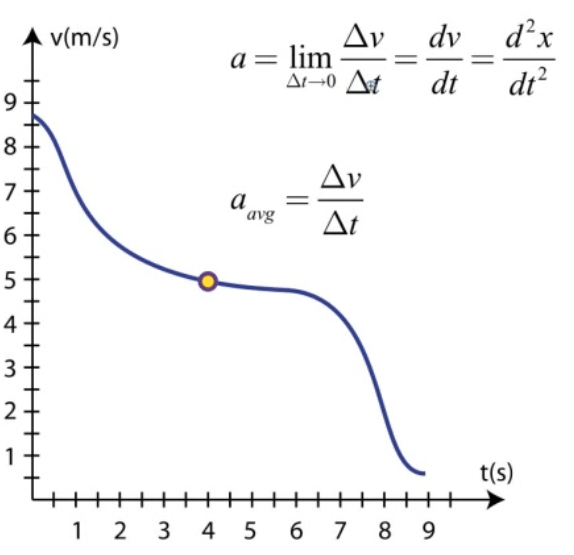# Position / Displacement

• An object's position is its location at a given point in time

•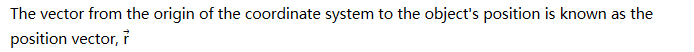•• Position and displacement are both vectors

•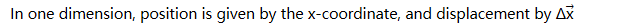# Average Speed

• Average speed is the distance traveled divided by the time interval

•• Average speed is a scalar, and is measured in meters/second

# Average Velocity

• Velocity is the rate at which position changes.

• Average velocity is the displacement during a time interval divided by the time interval

•• Average velocity is a vector, and is also measured in meter/second

# Acceleration

• Acceleration is the rate at which velocity changes

•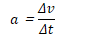• Acceleration is a vector

• Units are m/s2

# The Position Vector# Average Velocity# Instantaneous Velocity

• Average velocity observed over an infinitely small time interval provides instantons velocity

• Instantaneous velocity is the derivative of position with respect to time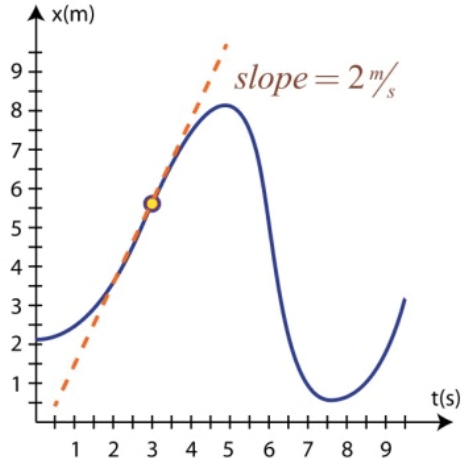• Area under the velocity-time graph is the displacement during that time interval# Acceleration

• Acceleration is the rate at which velocity changes